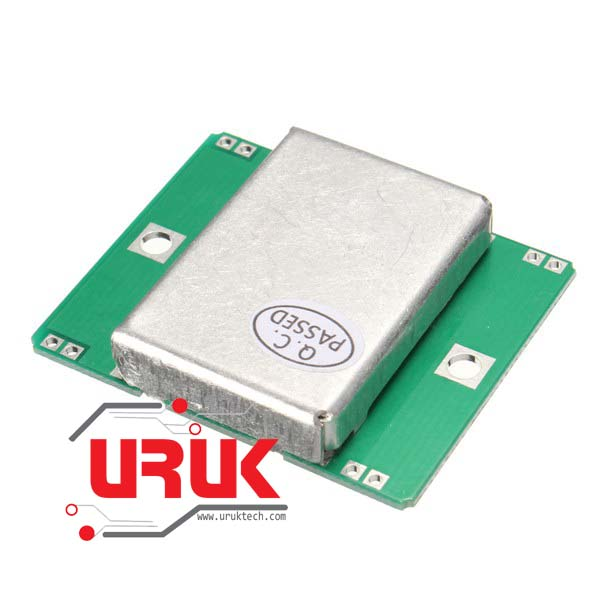# Doppler Radar Speed Detection using an HB100 10.525ghz radar gun.

The HB100 is a 10.525Ghz radar gun, normally found in automatic door openers. But it can be used for other things.

For most of the information I used to finally get this to work, I referred to the “Microwave Sensor Application Notes”

http://www.limpkin.fr/public/HB100/HB100_Microwave_Sensor_Application_Note.pdf

It contains all the information, radiation data, and circuit to amplify the output single.

The HB100 has an IF output frequency that is or should between 10hz and (say) 400hz.

It gives the formula to figure out Doppler frequency, which is

Fd = 2V(Ft/c)COS theta

Fd = Doppler Frequency, V = Velocity of the target, Ft = Transmit Frequency, c = speed of light (3 * 10^8 msec)

theta = the angle between the target moving direction adn the axis of the module.

IF the target is moving straight toward or away from HB100 (Ft = 10.525 Ghz) the formula is simplified to:

Fd = 19.49V (Velocity in km/hour) or 31.36V (V in mile per hour)

So we are going to use the simplest of the two, we know the doppler frequency, and we want to find the speed.

V = Fd/31.36 … What this really means thou, is for ever 31.36Hz will equal 1 MPH

So if the IF Frequency is 31.36 then the speed of the target is 1 MPH. Easy!

And a IF Frequency of say 400Hz would be about 13Mph if you round it up.

This was made even easier to find the frequency of the IF because I found a sketch that was a Frequency counter of sorts, and after the amplifier was built, I started to get some good speeds off the radar.

The FreqMeasure Library can be found here:

https://www.pjrc.com/teensy/td_libs_FreqMeasure.html

This was a hugh time saver, and it works very well.

You do have to approach the gun from head on, but it works and does a good job, and give speeds that I believe are very close to being right.  Over the next day or so I’ll try to get out on the road and see if I can get some real speed tests.

A new library and better results:

http://interface.khm.de/index.php/lab/experiments/frequency-measurement-library/

Found the above library from a link on the prjc website, this is apparently the library he based his library off of. What is interesting is I think I am getting better results from the original work then I was from the prjc libraries.

Used Items in This Tutorial:

A couple of things, the library seems to be using 3 pins for input, and a couple of resistors,  but I found that with this doppler setup really only one pin was needed (as it was before) however it’s pin 7, a resistor doesn’t appear to be needed.  The library doesn’t have any “examples” to speak of, and the code that is on the site contains many errors (Yup, I spotted a lot of errors before I even got started)  I am thinking the code was written in pure C and the library was written for the arduino (maybe even an old version of the IDE)  It hasn’t been updated since 2012.

Code:

SO the code I came up with:

#include “FreqPeriod.h”

double lfrq;

long int pp;

void setup() {

Serial.begin(9600);

FreqPeriod::begin();

Serial.println(“FreqPeriod Library Test”);

}

void loop() {

pp = FreqPeriod::getPeriod();

if (pp) {

Serial.print (“period: “);

Serial.print(pp);

Serial.print(“ 1/16us / frequency: “);

lfrq = 16000400.0 /pp;

Serial.print(lfrq);

Serial.print(“ Hz “);

Serial.print(lfrq/31.36);

Serial.println( “ Mph “);

}

}

This library is a lot faster then the FreqMeasure library (which seems to be taking an avg over a period of time and giving back a freq.) This one seems to just spit out freqs. So it seems like it’s a lot more sensitive to changes.  In fact as I approve the  doppler even from the back side of it, I get a change on the screen.  Good/Bad??? I’m not sure of the answer.

The speeds seem much more reasonable as well, and perhaps that is the math using the double integer, perhaps that is the fact that I am dividing the HZ / 31.36 (which is the real number to use)